# NCERT Solutions - Determinants, Exercise 4.6 JEE Notes | EduRev

## JEE : NCERT Solutions - Determinants, Exercise 4.6 JEE Notes | EduRev

The document NCERT Solutions - Determinants, Exercise 4.6 JEE Notes | EduRev is a part of the JEE Course Mathematics (Maths) Class 12.
All you need of JEE at this link: JEE

Determinants

Exercise 4.6

Question 1: Examine the consistency of the system of equations.
x + 2y = 2
2x + 3y = 3

The given system of equations is:
x + 2y = 2
2x + 3y = 3
The given system of equations can be written in the form of AX = B, where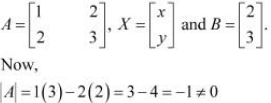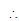A is non-singular.
Therefore, A−1 exists.
Hence, the given system of equations is consistent.

Question 2: Examine the consistency of the system of equations.
2x − y = 5 x
+ y = 4

The given system of equations is:
2x − y = 5 x
+ y = 4
The given system of equations can be written in the form of AX = B, where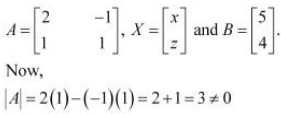A is non-singular.
Therefore, A−1 exists.
Hence, the given system of equations is consistent.

Question 3: Examine the consistency of the system of equations.
x + 3y = 5
2x + 6y = 8

The given system of equations is:
x + 3y = 5
2x + 6y = 8
The given system of equations can be written in the form of AX = B, where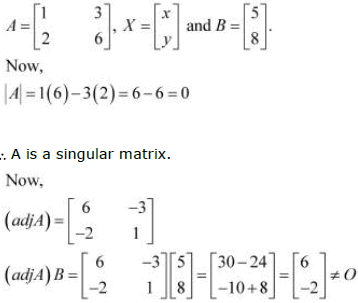Thus, the solution of the given system of equations does not exist. Hence, the system of equations is inconsistent.

Question 4: Examine the consistency of the system of equations.
x + y + z = 1

2x + 3y + 2z = 2

ax + ay + 2az = 4

The given system of equations is:
x + y + z = 1 2x
+ 3y + 2z = 2 ax
+ ay + 2az = 4
This system of equations can be written in the form AX = B, where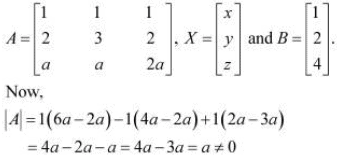A is non-singular.
Therefore, A−1 exists.
Hence, the given system of equations is consistent.

Question 5: Examine the consistency of the system of equations.
3x − y − 2z = 2
2y − z = −1
3x − 5y = 3

The given system of equations is:
3x − y − 2z = 2
2y − z = −1
3x − 5y = 3
This system of equations can be written in the form of AX = B, where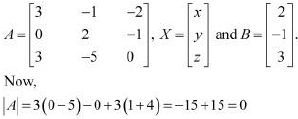A is a singular matrix.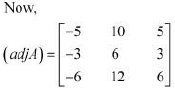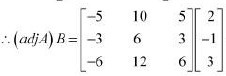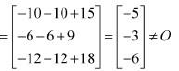Thus, the solution of the given system of equations does not exist. Hence, the system of equations is inconsistent.

Question 6: Examine the consistency of the system of equations.
5x − y + 4z = 5
2x + 3y + 5z = 2
5x − 2y + 6z = −1

The given system of equations is:
5x − y + 4z = 5
2x + 3y + 5z = 2
5x − 2y + 6z = −1
This system of equations can be written in the form of AX = B, where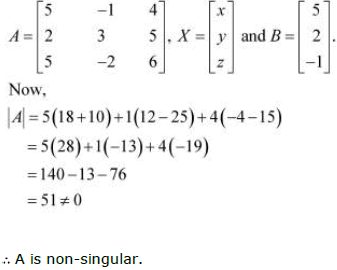Therefore, A−1 exists.
Hence, the given system of equations is consistent.

Question 7: Solve system of linear equations, using matrix method.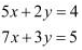The given system of equations can be written in the form of AX = B, where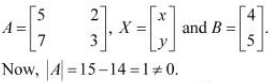Thus, A is non-singular. Therefore, its inverse exists.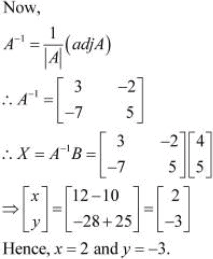Question 8: Solve system of linear equations, using matrix method.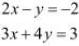Answer :The given system of equations can be written in the form of AX = B, where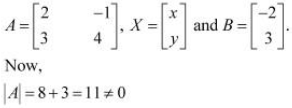Thus, A is non-singular. Therefore, its inverse exists.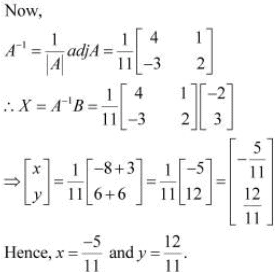Question 9: Solve system of linear equations, using matrix method.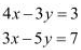Answer The given system of equations can be written in the form of AX = B, where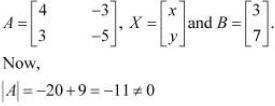Thus, A is non-singular. Therefore, its inverse exists.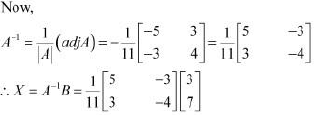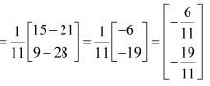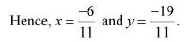Question 10: Solve system of linear equations, using matrix method.
5x + 2y = 3
3x + 2y = 5

Answer: The given system of equations can be written in the form of AX = B, where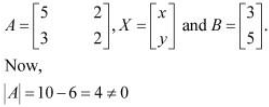Thus, A is non-singular. Therefore, its inverse exists.

Question 11: Solve system of linear equations, using matrix method.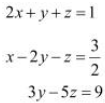Answer: The given system of equations can be written in the form of AX = B, where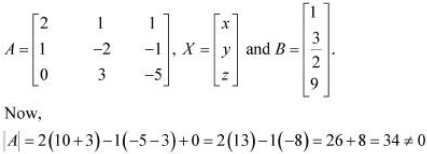Thus, A is non-singular. Therefore, its inverse exists.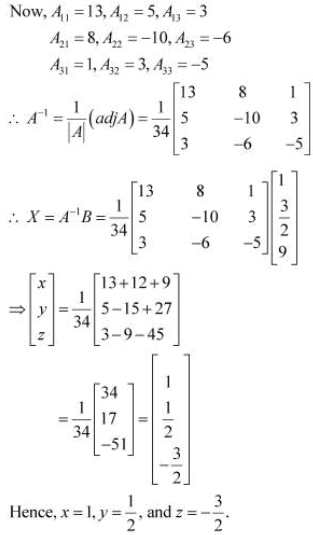Question 12: Solve system of linear equations, using matrix method.
x − y + z = 4

2x + y − 3z = 0

x + y + z = 2

Answer: The given system of equations can be written in the form of AX = B, where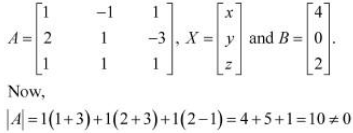Thus, A is non-singular. Therefore, its inverse exists.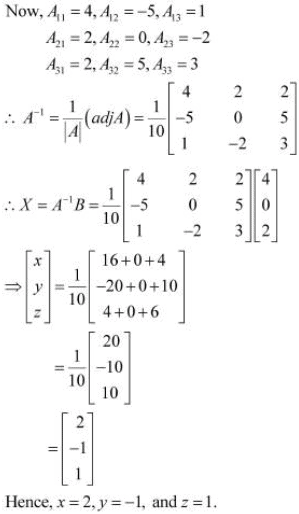Question 13: Solve system of linear equations, using matrix method.
2x + 3y + 3z = 5
x − 2y + z = −4
3x − y − 2z = 3

Answer: The given system of equations can be written in the form AX = B, where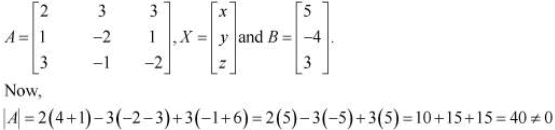Thus, A is non-singular. Therefore, its inverse exists.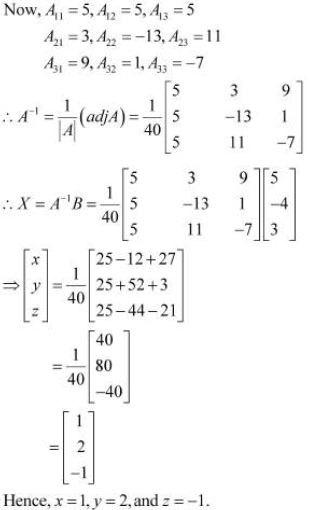Question 14: Solve system of linear equations, using matrix method.

x − y + 2z = 7
3x + 4y − 5z = −5
2x − y + 3z = 12

Answer: The given system of equations can be written in the form of AX = B, where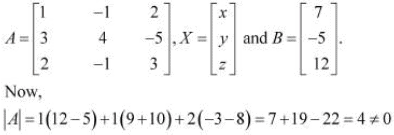Thus, A is non-singular. Therefore, its inverse exists.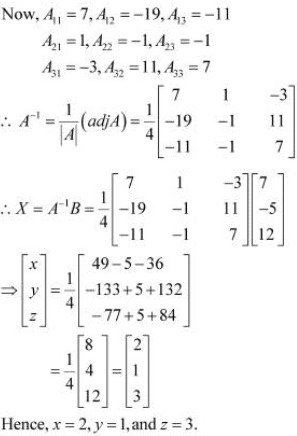Question 15:

If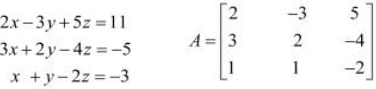find A−1. Using A−1 solve the system of equations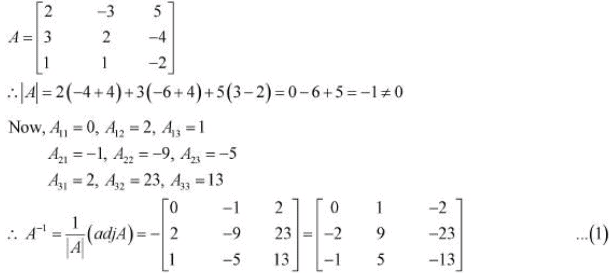Now, the given system of equations can be written in the form of AX = B, where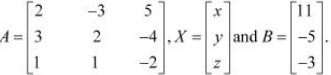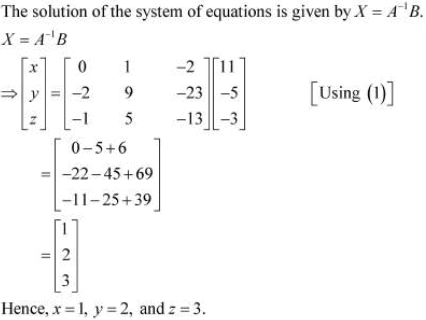Question 16: The cost of 4 kg onion, 3 kg wheat and 2 kg rice is Rs 60. The cost of 2 kg onion, 4 kg wheat and 6 kg rice is Rs 90. The cost of 6 kg onion 2 kg wheat and 3 kg rice is Rs 70. Find cost of each item per kg by matrix method.

Let the cost of onions, wheat, and rice per kg be Rs x, Rs y,and Rs z respectively.
Then, the given situation can be represented by a system of equations as: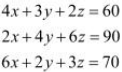This system of equations can be written in the form of AX = B, where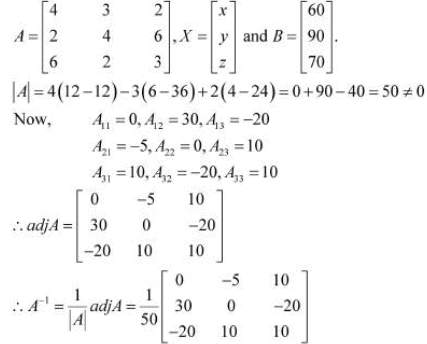Now,
X = A−1 B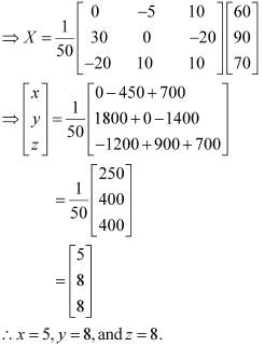Hence, the cost of onions is Rs 5 per kg, the cost of wheat is Rs 8 per kg, and the cost of rice is Rs 8 per kg.

Offer running on EduRev: Apply code STAYHOME200 to get INR 200 off on our premium plan EduRev Infinity!

## Mathematics (Maths) Class 12

209 videos|222 docs|124 tests

,

,

,

,

,

,

,

,

,

,

,

,

,

,

,

,

,

,

,

,

,

,

,

,

;